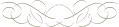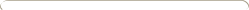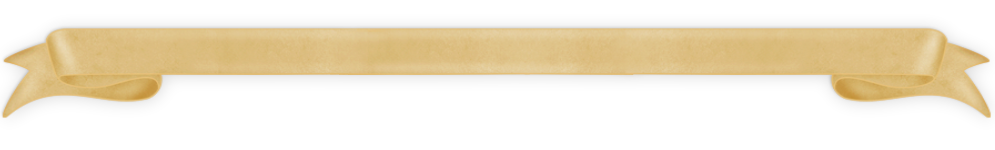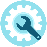﻿ Facit 1113Details:

This electronic calculator was produced (and I guess also developed) by Sharp.  It contains a single Hitachi integrated circuit and a VFD display.  The position of the  decimal point is determined automatically.  The number of digits can be set to 0, 2, 4 or max.   If desired a memory totals all calculation results.

The sign of the calculated result is shown in the utmost right of the display.  At that same position an overflow is shown by a “C” or “E” while a dot at that position indicates that the memory contains a number.

Usage:

Enter a number, then pushing  “ += “ adds the number to the result register which is shown in the display.

- =“ subtracts in the same way.

Multiplication:

Enter the 1st number, push the “X” key, enter the 2nd number, push the “+=” or the  “- =“  key.

Division:

Similar to multiplication using the “:” key.

Usage of the % key:

To add a percentage to a number, enter the number, then multiply it with the percentage using the “X” key, then press the “%” key. The display shows the calculated percentage.  Pushing the “+=” key adds the increase to the number. The display shows the end result.

Example:  22  x  7 %  +=  23.54

Function of some keys, switches etc.

• The upper switches determine special operations:
• :      automatically sums results of calculations  in memory
• K:      uses the displayed number as first operand
• 5/4:   rounds as usual
• 0 | 2 | 4 :  determines the number of digits behind the decimal point
• C”  clears the machine except memory
• CE”  clears last entry
• RM/CM”  reads memory to input and clears memoryClick to view a full size picture (opens in a new tab)

Serial number:

Build in:

Functions:

Power:

Market-introduction:

Production until:

Price:

617 0656

Probably early 1976